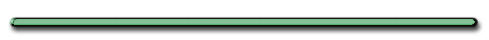Momentum

I. Momentum - the property of a moving object that makes it difficult to stop."Which is tougher to stop, a bowling ball or  a baseball?"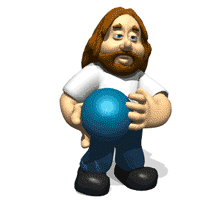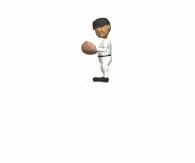It Depends ....If the baseball had a much larger velocity, it  would be harder to stop.

(technically, product of mass and velocity)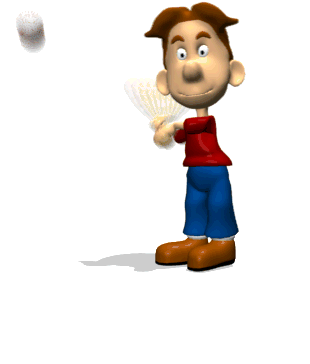Momentum = Mass x Velocity

p = mv

(kgm/s)  (kg)(m/s)

a) vector quantity

b) p has same direction as velocity

c) units - kgm/s

Momentum helps us
understand collisions

[Flash]

Kinetic Energy is conserved in perfectly elastic collisions

 Perfectly Elastic Collision?

No

The ball's energy is lost in the collision with the floor. It does not return its original position."Could a moving baseball have the same momentum as moving bowling ball?""Yes. p = mv. The baseball's velocity would have to be many  times faster."

1) Relationship between force and momentum

When a force acts on a body it
changes its' momentum.

Mathematical Relationship, F, p

p = mv

so ...

Δp = mΔv

Fnet = ma

so ...

 Fnet = m Δv t

(Cross Multiply)

Fnett = mΔv

Since ...

Δp = mΔv

Then ...

Fnett = Δp

Force is the time rate
of change in momentum

 Fnet = Δp t

Δp = FtThe change in momentum that an unbalanced force produces on an object depends on ....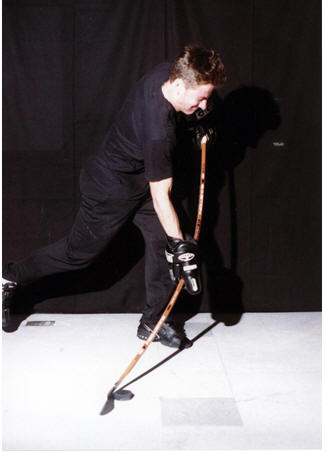... how long the force acts on a body."Could a female golfer EVER change the momentum of a golf ball more than a male golfer?"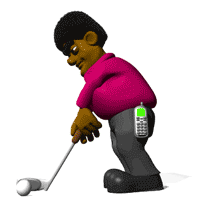Yes. She can compensate for a smaller force
by employing a good 'follow through'

2)  Impulse

 J = Fnett (Ns) or (kg•m/s)

Combining all the equations

 * J = Fnett = mΔv =Δp *

Given this equation, what is another unit for momentum besides kgm/s?

 * J = Fnett = mΔv =Δp *

Ns